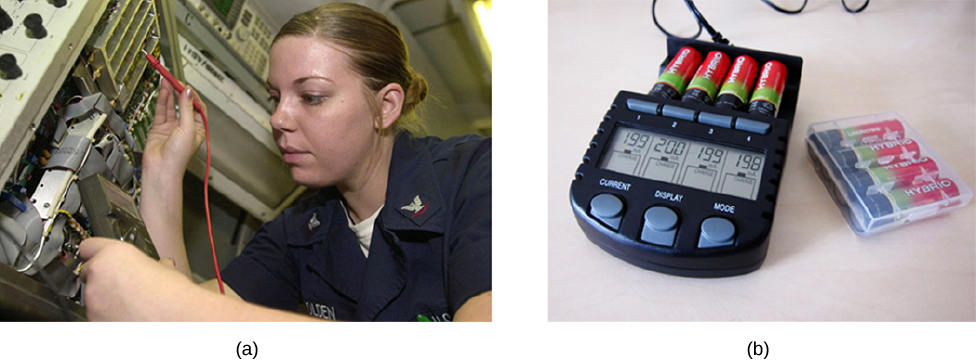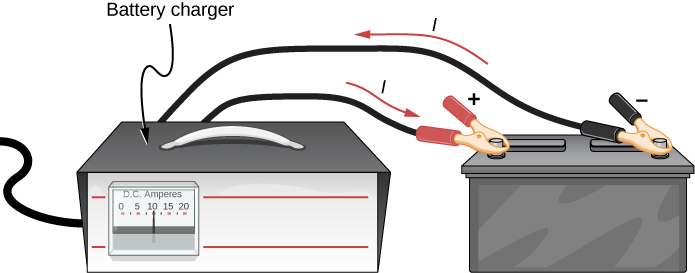# 10.1 Electromotive force  (Page 5/11)

 Page 5 / 11

## Significance

The internal resistance of a battery can increase for many reasons. For example, the internal resistance of a rechargeable battery increases as the number of times the battery is recharged increases. The increased internal resistance may have two effects on the battery. First, the terminal voltage will decrease. Second, the battery may overheat due to the increased power dissipated by the internal resistance.

Check Your Understanding If you place a wire directly across the two terminal of a battery, effectively shorting out the terminals, the battery will begin to get hot. Why do you suppose this happens?

If a wire is connected across the terminals, the load resistance is close to zero, or at least considerably less than the internal resistance of the battery. Since the internal resistance is small, the current through the circuit will be large, $I=\frac{\epsilon }{R+r}=\frac{\epsilon }{0+r}=\frac{\epsilon }{r}.$ The large current causes a high power to be dissipated by the internal resistance $\left(P={I}^{2}r\right)$ . The power is dissipated as heat.

## Battery testers

Battery testers , such as those in [link] , use small load resistors to intentionally draw current to determine whether the terminal potential drops below an acceptable level. Although it is difficult to measure the internal resistance of a battery, battery testers can provide a measurement of the internal resistance of the battery. If internal resistance is high, the battery is weak, as evidenced by its low terminal voltage.Battery testers measure terminal voltage under a load to determine the condition of a battery. (a) A US Navy electronics technician uses a battery tester to test large batteries aboard the aircraft carrier USS Nimitz . The battery tester she uses has a small resistance that can dissipate large amounts of power. (b) The small device shown is used on small batteries and has a digital display to indicate the acceptability of the terminal voltage. (credit a: modification of work by Jason A. Johnston; credit b: modification of work by Keith Williamson)

Some batteries can be recharged by passing a current through them in the direction opposite to the current they supply to an appliance. This is done routinely in cars and in batteries for small electrical appliances and electronic devices ( [link] ). The voltage output of the battery charger must be greater than the emf of the battery to reverse the current through it. This causes the terminal voltage of the battery to be greater than the emf, since $V=\epsilon -Ir$ and I is now negative.A car battery charger reverses the normal direction of current through a battery, reversing its chemical reaction and replenishing its chemical potential.

It is important to understand the consequences of the internal resistance of emf sources, such as batteries and solar cells, but often, the analysis of circuits is done with the terminal voltage of the battery, as we have done in the previous sections. The terminal voltage is referred to as simply as V , dropping the subscript “terminal.” This is because the internal resistance of the battery is difficult to measure directly and can change over time.

calculate ideal gas pressure of 0.300mol,v=2L T=40°c
what is principle of superposition
what are questions that are likely to come out during exam
what is electricity
watt is electricity.
electricity ka full definition with formula
Jyoti
If a point charge is released from rest in a uniform electric field will it follow a field line? Will it do so if the electric field is not uniform?
Maxwell's stress tensor is
Yes
doris
neither vector nor scalar
Anil
if 6.0×10^13 electrons are placed on a metal sphere of charge 9.0micro Coulombs, what is the net charge on the sphere
18.51micro Coulombs
ASHOK
Is it possible to find the magnetic field of a circular loop at the centre by using ampere's law?
Is it possible to find the magnetic field of a circular loop at it's centre?
yes
Brother
The density of a gas of relative molecular mass 28 at a certain temperature is 0.90 K kgmcube.The root mean square speed of the gas molecules at that temperature is 602ms.Assuming that the rate of diffusion of a gas in inversely proportional to the square root of its density,calculate the density of
A hot liquid at 80degree Celsius is added to 600g of the same liquid originally at 10 degree Celsius. when the mixture reaches 30 degree Celsius, what will be the total mass of the liquid?
Under which topic
doris
what is electrostatics
Study of charges which are at rest
himanshu
Explain Kinematics
Two equal positive charges are repelling each other. The force on the charge on the left is 3.0 Newtons. Using your notes on Coulomb's law, and the forces acting on each of the charges, what is the force on the charge on the right?
Using the same two positive charges, the left positive charge is increased so that its charge is 4 times LARGER than the charge on the right. Using your notes on Coulomb's law and changes to the charge, once the charge is increased, what is the new force of repulsion between the two positive charges?
Nya
A mass 'm' is attached to a spring oscillates every 5 second. If the mass is increased by a 5 kg, the period increases by 3 second. Find its initial mass 'm'
a hot water tank containing 50,000g of water is heated by an electric immersion heater rated at 3kilowatt,240volt, calculate the currentBy Edgar DelgadoBy Joli JuliannaBy John GabrieliBy OpenStaxBy Yacoub JayoghliBy Gerr ZenBy OpenStaxByBy OpenStaxBy Dakota Bocan